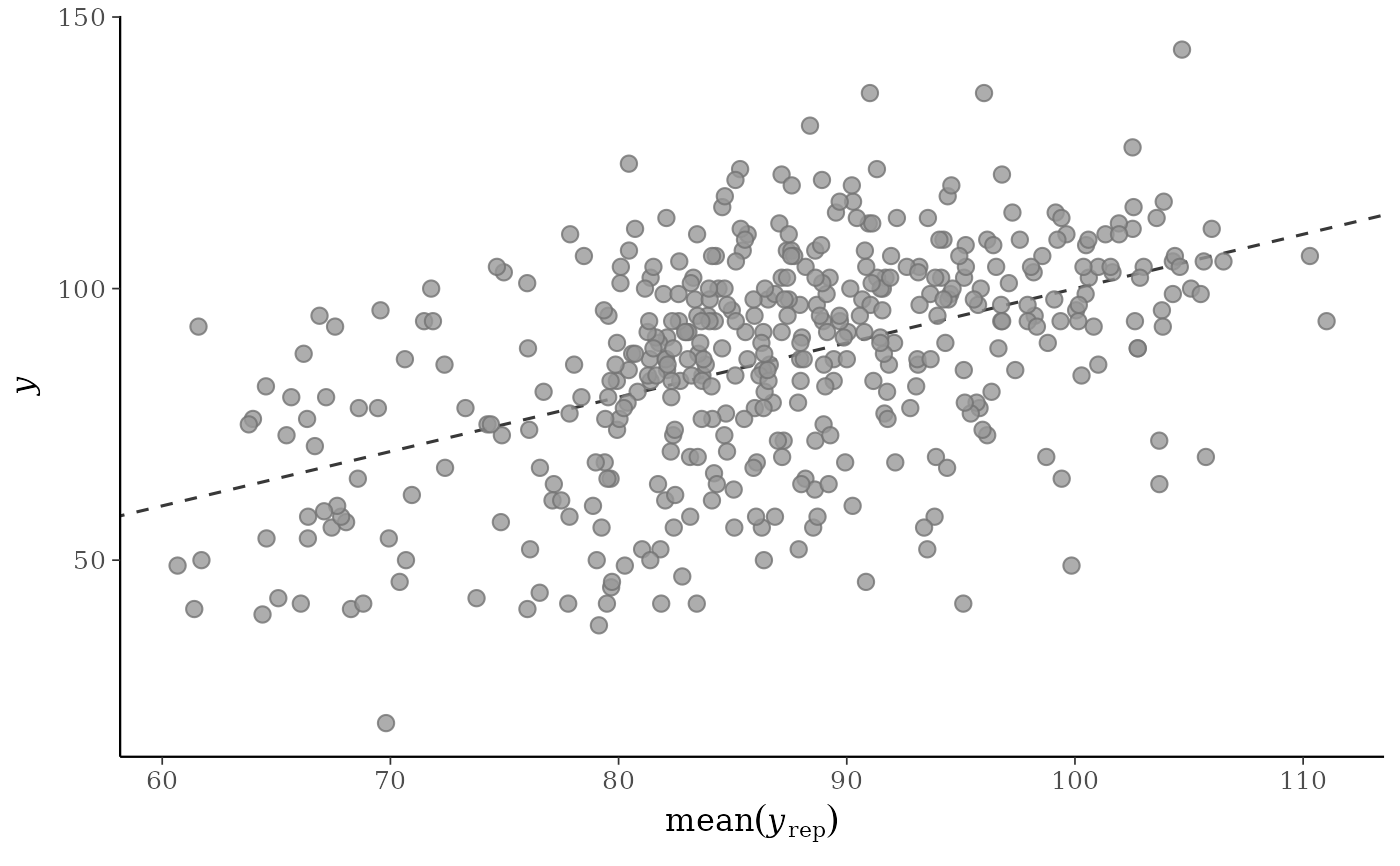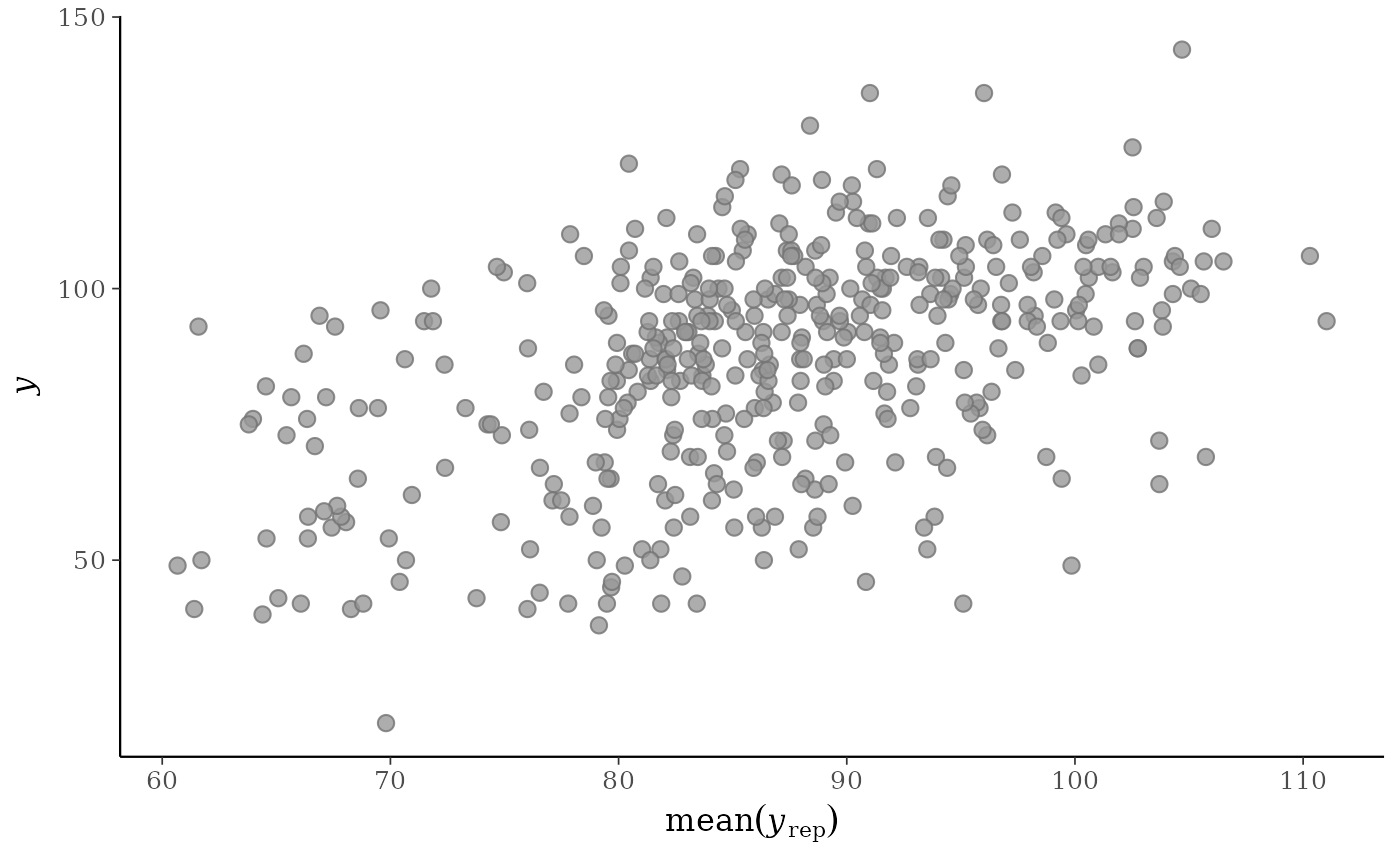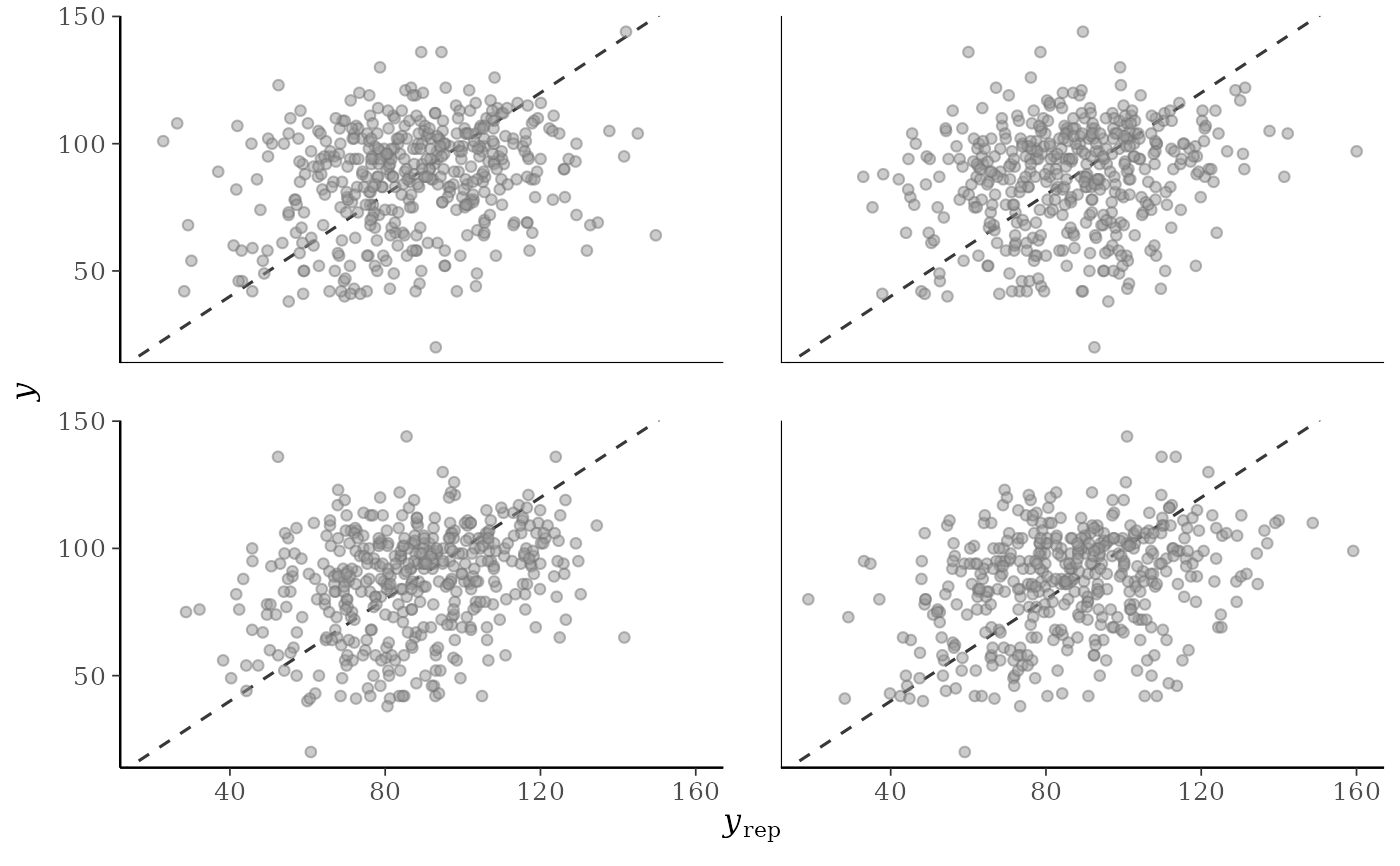Scatterplots of the observed data y vs. simulated/replicated data yrep from the posterior predictive distribution. See the Plot Descriptions and Details sections, below.

ppc_scatter(y, yrep, ..., size = 2.5, alpha = 0.8)

ppc_scatter_avg(y, yrep, ..., size = 2.5, alpha = 0.8)

ppc_scatter_avg_grouped(y, yrep, group, ..., size = 2.5, alpha = 0.8)

## Arguments

y A vector of observations. See Details. An $$S$$ by $$N$$ matrix of draws from the posterior predictive distribution, where $$S$$ is the size of the posterior sample (or subset of the posterior sample used to generate yrep) and $$N$$ is the number of observations (the length of y). The columns of yrep should be in the same order as the data points in y for the plots to make sense. See Details for additional instructions. Currently unused. Arguments passed to ggplot2::geom_point() to control the appearance of the points. A grouping variable (a vector or factor) the same length as y. Each value in group is interpreted as the group level pertaining to the corresponding value of y.

## Value

A ggplot object that can be further customized using the ggplot2 package.

## Details

For Binomial data, the plots will typically be most useful if y and yrep contain the "success" proportions (not discrete "success" or "failure" counts).

## Plot Descriptions

ppc_scatter()

For each dataset (row) in yrep a scatterplot is generated showing y against that row of yrep. For this plot yrep should only contain a small number of rows.

ppc_scatter_avg()

A scatterplot of y against the average values of yrep, i.e., the points (mean(yrep[, n]), y[n]), where each yrep[, n] is a vector of length equal to the number of posterior draws.

ppc_scatter_avg_grouped()

The same as ppc_scatter_avg(), but a separate plot is generated for each level of a grouping variable.

Gelman, A., Carlin, J. B., Stern, H. S., Dunson, D. B., Vehtari, A., and Rubin, D. B. (2013). Bayesian Data Analysis. Chapman & Hall/CRC Press, London, third edition. (Ch. 6)

Other PPCs: PPC-censoring, PPC-discrete, PPC-distributions, PPC-errors, PPC-intervals, PPC-loo, PPC-overview, PPC-test-statistics

## Examples

y <- example_y_data()
yrep <- example_yrep_draws()
p1 <- ppc_scatter_avg(y, yrep)
p1p2 <- ppc_scatter(y, yrep[20:23, ], alpha = 0.5, size = 1.5)
p2# give x and y axes the same limits
lims <- ggplot2::lims(x = c(0, 160), y = c(0, 160))
p1 + limsp2 + lims#> Warning: Removed 1 rows containing missing values (geom_point).group <- example_group_data()
ppc_scatter_avg_grouped(y, yrep, group, alpha = 0.7) + lims